Power Plant

# Power Plant - Mechanical Engineering

Test Description

## 25 Questions MCQ Test GATE Mechanical (ME) 2023 Mock Test Series - Power Plant

Power Plant for Mechanical Engineering 2023 is part of GATE Mechanical (ME) 2023 Mock Test Series preparation. The Power Plant questions and answers have been prepared according to the Mechanical Engineering exam syllabus.The Power Plant MCQs are made for Mechanical Engineering 2023 Exam. Find important definitions, questions, notes, meanings, examples, exercises, MCQs and online tests for Power Plant below.
Solutions of Power Plant questions in English are available as part of our GATE Mechanical (ME) 2023 Mock Test Series for Mechanical Engineering & Power Plant solutions in Hindi for GATE Mechanical (ME) 2023 Mock Test Series course. Download more important topics, notes, lectures and mock test series for Mechanical Engineering Exam by signing up for free. Attempt Power Plant | 25 questions in 75 minutes | Mock test for Mechanical Engineering preparation | Free important questions MCQ to study GATE Mechanical (ME) 2023 Mock Test Series for Mechanical Engineering Exam | Download free PDF with solutions
 1 Crore+ students have signed up on EduRev. Have you?
Power Plant - Question 1

### Common data for 1 & 2 Air enters the compressor of an ideal air standard Brayton cycle at 100 kPa, 200C and the pressure ratio across the compressor is 12:1.  Flow rate of air is 10 kg/s and maximum temperature in cycle is 11000C. Q. Compressor Power input is:-

Detailed Solution for Power Plant - Question 1

P2/P1 = 12.

then T2= (12)^{0..4/1.4} × 293 = 595.94K.

Then in ideal brayton cycle work done by compressor is =(h2- h1)= m×Cp× (T2-T1)= 10×1.005× (595.94-293) = 3044.54 KW = 3.04 MW

Power Plant - Question 2

### Common data for 1 & 2 Air enters the compressor of an ideal air standard Brayton cycle at 100 kPa, 200C and the pressure ratio across the compressor is 12:1. Flow rate of air is 10 kg/s and maximum temperature in cycle is 11000C. Q. Turbine Power output is:-

Detailed Solution for Power Plant - Question 2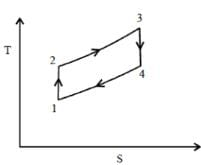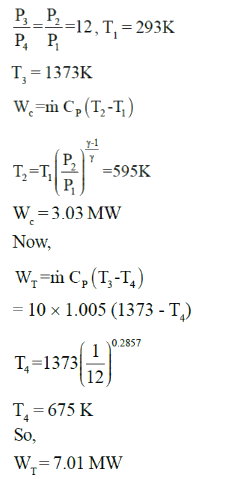Power Plant - Question 3

### Common data for 3 & 4 Brayton cycle has compression ratio of 16:1. Cycle produces 14 MW. Inlet state is 100 kPa, 170C. Heat added in combustion is 960 kJ/kg. Q. Highest temperature will be:-

Power Plant - Question 4

Common data for 3 & 4

Brayton cycle has compression ratio of 16:1. Cycle produces 14 MW. Inlet state is 100 kPa, 170C. Heat added in combustion is 960 kJ/kg.

Q. Mass flow rate of air will be :-

Detailed Solution for Power Plant - Question 4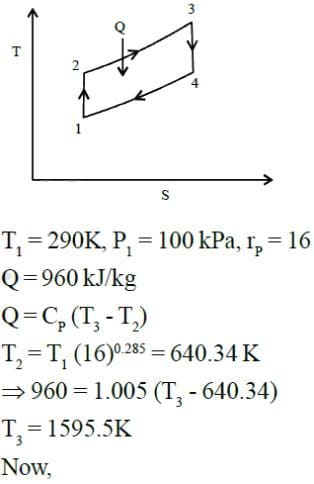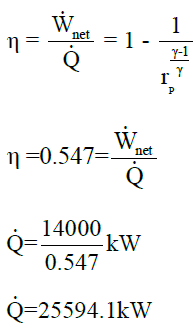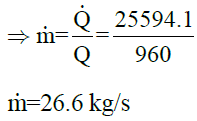Power Plant - Question 5

Temperature limits in an ideal air standard Brayton cycle are 300 K and 1500K. Pressure ratio is such that it maximises the net work developed by the cycle per unit mass of air flow. Thermal efficiency of cycle is:-

Detailed Solution for Power Plant - Question 5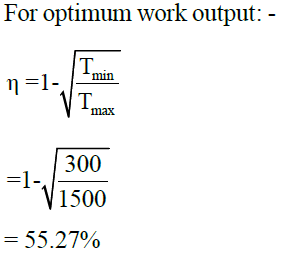Power Plant - Question 6

Compressor inlet temperature in ideal Brayton cycle is T1, turbine inlet temperature is T3. For maximum net work developed per unit mass of air flow, the temperature T2 at the compressor exit will be:-

Detailed Solution for Power Plant - Question 6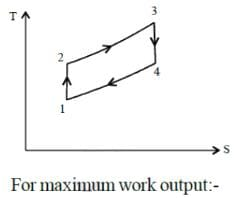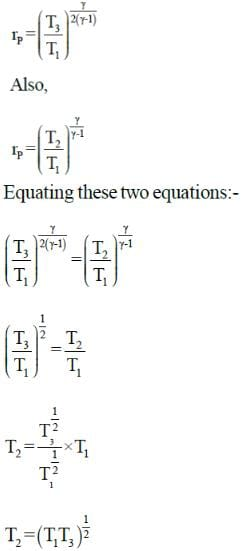Power Plant - Question 7

Brayton cycle operates between temperature limits of 270C and 7270C. Air is the working fluid. Maximum and minimum cycle pressure are 2000 kPa and 100 kPa respectively. Net work produced by this cycle is:-

Detailed Solution for Power Plant - Question 7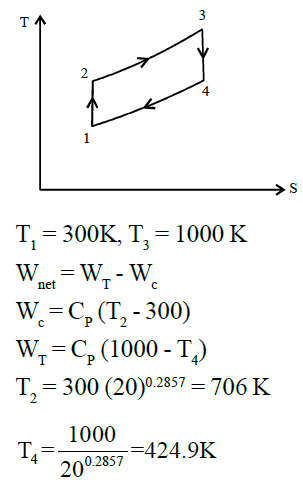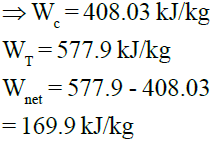Power Plant - Question 8

A simple Brayton Cycle has a pressure ratio of 10. The air at the beginning of compressor is at 70 kPa and 00C. Heat is added to the cycle at a rate of 500 kW and air passes at the rate of 1kg/s.
Power produced by this cycle is:-

Detailed Solution for Power Plant - Question 8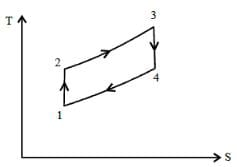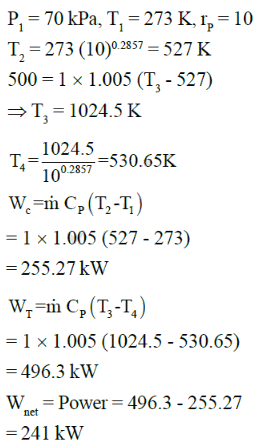Power Plant - Question 9

Brayton cycle operates between temperature limits of 300K and 1600 K. Power output is 100 MW.
The compressor pressure ratio is 14 to 1.

Thermal efficiency of cycle is:-

Detailed Solution for Power Plant - Question 9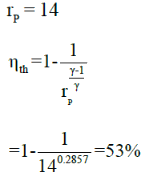Power Plant - Question 10

Carnot cycle operates between pressure and temperature limits of 20 kPa to 2000 kPa and 300 K to 900K respectively. If cycle executed in a closed system with 0.003 kg of air. Net work output per cycle will be:-

Detailed Solution for Power Plant - Question 10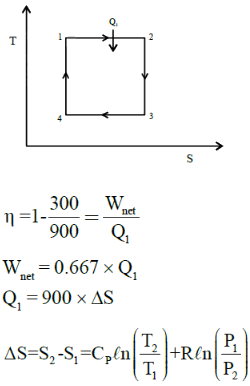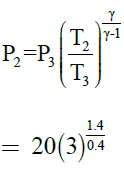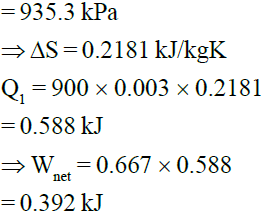Power Plant - Question 11

Carnot cycle uses heluim (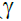= 1.667) as working fluid and rejects heat to lake at 150C. If thermal efficiency of cycle is to be 50%, the compression ratio will be:

Detailed Solution for Power Plant - Question 11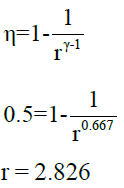Power Plant - Question 12

Ideal gas carnot cycle receives heat at 10270C. The cycle is repeated 1500 times per minute and has compression ratio of 12. Device produces power of 500 kW, the amount of heat supplied per cycle must be:-

Detailed Solution for Power Plant - Question 12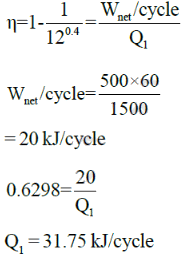Power Plant - Question 13

Common data for 13 & 14
Simple ideal Rankine Cycle has water as working Fluid. Cycle operates between 15 MPa in boiler and 100 kPa in condenser. Saturated steam enters turbine and steam quality at outlet of turbine is 70%

Q. Thermal efficiency of cycle is:-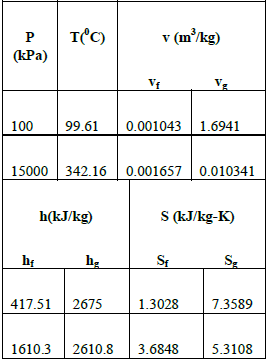Power Plant - Question 14

Common data for 13 & 14
Simple ideal Rankine Cycle has water as working Fluid. Cycle operates between 15 MPa in boiler and 100 kPa in condenser. Saturated steam enters turbine and steam quality at outlet of turbine is 70%Q. Isentropic efficiency of turbine is:-

Detailed Solution for Power Plant - Question 14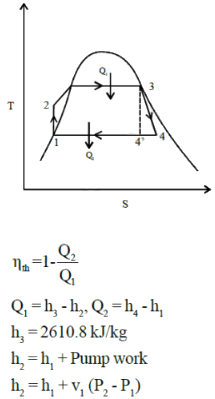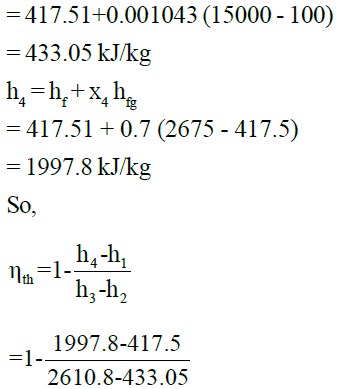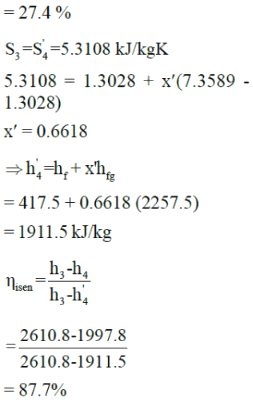Power Plant - Question 15

High pressure in Rankine cycle is determined in :-

Detailed Solution for Power Plant - Question 15

High pressure is determined by pump whereas low pressure is determined as saturation pressure for temperature. We can cool to in condenser

Power Plant - Question 16

Superheated vapour enters the turbine at 80bar, 4800C in an ideal Rankine cycle and condenser pressure in cycle is 0.08 bar. Net power output of cycle is 100 MW.

Consider the following table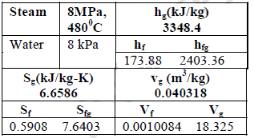Rate of heat transfer to working fluid passing through steam generator (in MW) is:-

Detailed Solution for Power Plant - Question 16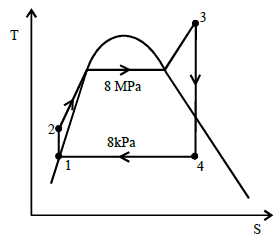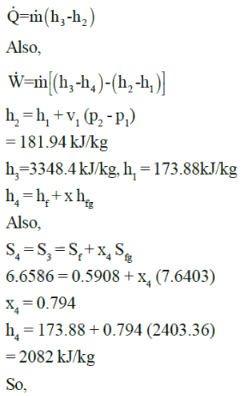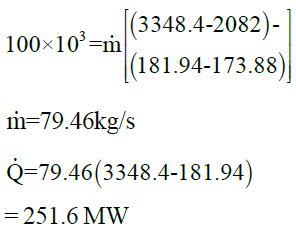Power Plant - Question 17

Common data for 17 & 18
In ideal Rankine cycle, steam enters turbine at 30 MPa, 5500C and exit at a pressure of 5kPa. It enters
condenser and exits as saturated water. Pump feeds back the water to boiler.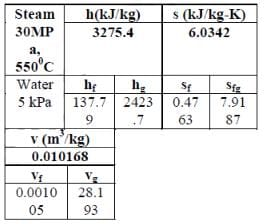Q. Steam quality at turbine exit is:-

Power Plant - Question 18

Common data for 17 & 18
In ideal Rankine cycle, steam enters turbine at 30 MPa, 5500C and exit at a pressure of 5kPa. It enters
condenser and exits as saturated water. Pump feeds back the water to boiler.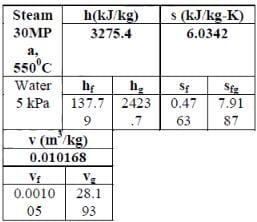Q. Thermal efficiency of cycle is:-

Detailed Solution for Power Plant - Question 18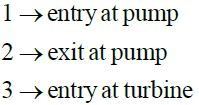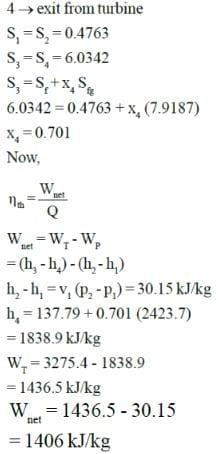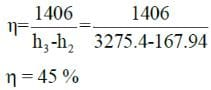Power Plant - Question 19

Simple ideal Rankine cycle use water as working fluid and operates between pressure limits of 3MPa in
boiler (h = 3115.5kJ/kg) and 30 kPa in condenser (hf = 289.27 kJ/kg, hfg = 2335.3 kJ/kg, v = 0.001022 m3/kg). If quality at exit of turbine cannot be less than 85%, maximum thermal efficiency of cycle is :-

Detailed Solution for Power Plant - Question 19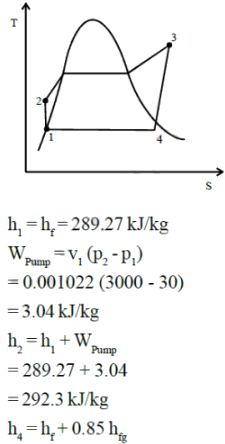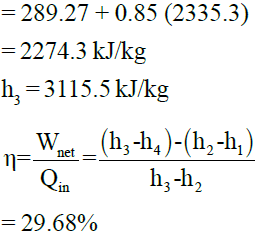Power Plant - Question 20

Consider a simple ideal Rankine cycle. If condenser pressure is lowered while keeping turbine inlet state the same.

Detailed Solution for Power Plant - Question 20

Area under the curve representing condenser decreases, so amount of heat rejected will decrease.

Power Plant - Question 21

Simple ideal Brayton cycle works between temperature limits of 300C and 8270C. Pressure ratio is 18 and
minimum cycle pressure is 100 kPa. Efficiency of this cycle is:-

(use standard value for air)

Detailed Solution for Power Plant - Question 21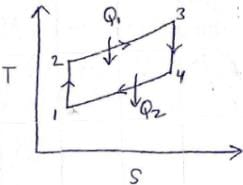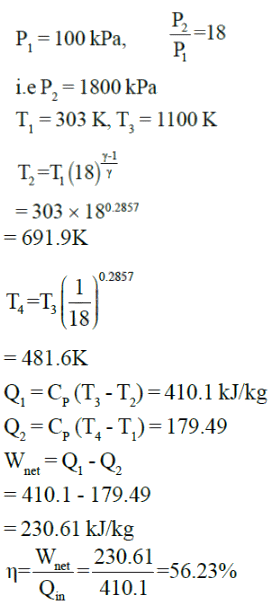Power Plant - Question 22

In a simple Brayton cycle, air has a pressure and temperature of 90 kPa and 100C before compression. It
has a pressure ratio of 10. Mass flow rate of air is 2 kg/s. Value of power produced by cycle is (heat is
added at rate of 600 kW):-

Detailed Solution for Power Plant - Question 22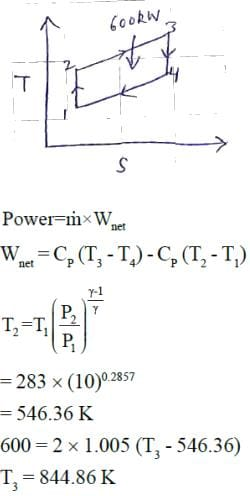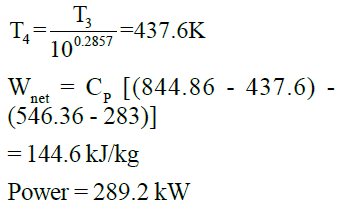Power Plant - Question 23

Thermal efficiency in previous question is given by :-

Detailed Solution for Power Plant - Question 23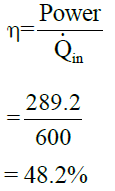Power Plant - Question 24

Maximum and minimum temperature in air standard gas turbine cycle are 1000 K and 200K. For maximum net work output, value of pressure ratio is:-

Detailed Solution for Power Plant - Question 24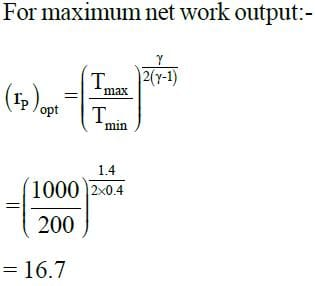Power Plant - Question 25

The isentropic enthalpy drop in moving blade is two-third of the isentropic enthalpy drop in fixed blades of a turbine. The degree of reaction will be

Detailed Solution for Power Plant - Question 25

Degree of reaction = isentropic enthalpy drop in moving blade/(isentropic enthalpy drop in fixed blade+isentropic enthalpy drop in moving blade).

= (2/3) / [1+(2/3)].

= (2/3) / (5/3),

= 2/5,

DOR = 0.4

## GATE Mechanical (ME) 2023 Mock Test Series

27 docs|243 tests(Scan QR code)